# SAT Math Multiple Choice Question 903: Answer and Explanation

### Test Information

Question: 903

3. Professors Lambert and Stassen team-teach an advanced psychology class. Their studentsrecently took their final exams, and the professors are preparing to grade them. ProfessorStassen predicts that, if she graded all the exams, she would take 50% more time thanProfessor Lambert would if he graded all the exams. They estimate that it will takethem 40 hours to grade all the exams if they grade together. Which of the followingequations best represents the situation described?

• A.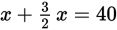• B.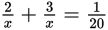• C.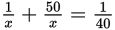• D.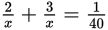Strategic Advice: When building an equation that describes how much work two people can do together versus independently, remember the additive nature of rates and the equation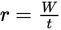.
Getting to the Answer: Let x represent Professor Lambert. If W (the work) represents the job of grading the exams and there is one job to complete, then Professor Lambert's grading speed (his rate) is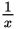. Professor Stassen's solo grading time is 50% greater than Professor Lambert's, so her rate is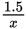. Working together, the two would take 40 hours to grade all the exams, which is a rate of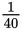exams per hour. Add the individual rates together and set them equal to the combined rate: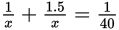. None of the answer choices contain a decimal in the numerator, so multiply your equation by 2 to eliminate the 1.5. The result is, which matches (B).